# CSS Text Formatting

The CSS text formatting properties are used to format text, style the text and perform different types of manipulations like word spacing, alignment, justification, and text transformation.

Syntax:

The Syntax to write this property:

```Selector {
property-name : /*value*/
}```

### CSS Text Formatting Properties:

These are the following text formatting properties.

• Text Color: This property is used to set the color of the text and the color can be set by using a color name like “red”, hex value “#ff0000”, or by its RGB value “rgb(255,0,0)”;
• text-align: This property in CSS is used to specify the horizontal alignment of text in an element inside a block element or table-cell box.
•  text-align-last: It is used to set the last line of the paragraph just before the line break. It sets the alignment of all the last lines occurring in the element in which the text-align-last property is applied.
• text-decoration: text-decoration property is used to “decorate ” the content of the text.
• text-decoration-color: It is used to set the color of the decorations (overlines, underlines, and line-throughs) over the text.
• text-decoration-line: It is used to set the various kinds of text decorations. this may include many values such as underline, overline, line-through, etc.
• text-decoration-style: This property is used to set the text-decoration of the element. It is the combination of the text-decoration-line and text-decoration-color properties.
• text-indent: It is used to indent the first line of the paragraph and the size can be in px, cm, pt.
• text-justify: This property is used to set the text-align to justify. It spreads the words into complete lines.
• text-overflow: This property of text formatting specify that some text has overflown and is hidden from the view.
• text-transform: It is used to control the capitalization of the text.
• letter-spacing: This property is used to specify the space between the characters of the text.
• line-height: It is used to set the space between the lines.
• direction: This property is used to set the direction of the text.
• word-spacing: It is used to specify the space between the words of the line.

Example 1: This example illustrates the use of text color property.

## html

 `` `<``html``>`   `<``head``>` `    ``<``style``>` `        ``h1 {` `            ``color: red;` `        ``}`   `        ``h2 {` `            ``color: green;` `        ``}` `    ``` ``   `<``body``>` `    ``<``h1``>` `        ``GEEKS FOR GEEKS` `    ``` `    ``<``h2``>` `        ``TEXT FORMATTING` `    ``` ``   ``

Output: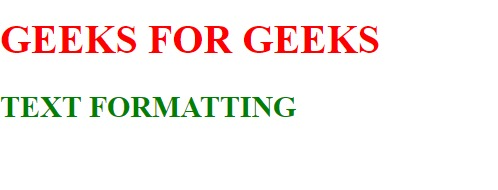Example 2: This example illustrates the use of the text-align property.

## html

 `` `<``html``>`   `<``head``>` `    ``<``style``>` `        ``h1 {` `            ``color: red;` `            ``text-align: center;` `        ``}`   `        ``h2 {` `            ``color: green;` `            ``text-align: left;` `        ``}` `    ``` ``   `<``body``>` `    ``<``h1``>` `        ``GEEKS FOR GEEKS` `    ``` `    ``<``h2``>` `        ``TEXT FORMATTING` `    ``` ``   ``

Output:Example 3: This example illustrates the use of the text-decoration property.

## html

 `` `<``html``>`   `<``head``>` `    ``<``style``>` `        ``h1 {` `            ``color: red;` `            ``text-decoration: underline;` `        ``}` `    ``` ``   `<``body``>` `    ``<``h1``>` `        ``GEEKS FOR GEEKS` `    ``` `    ``<``h2``>` `        ``TEXT FORMATTING` `    ``` ``   ``

Output: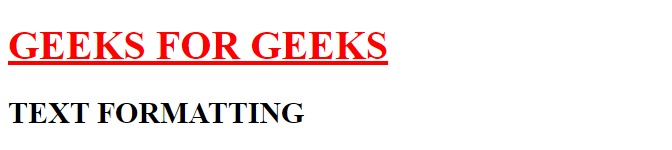Example 4: This example illustrates the use of the text-transform property.

## html

 `` `<``html``>`   `<``head``>` `    ``<``style``>` `        ``h2 {` `            ``text-transform: lowercase;` `        ``}` `    ``` ``   `<``body``>` `    ``<``h1``>` `        ``GEEKS FOR GEEKS` `    ``` `    ``<``h2``>` `        ``TEXT FORMATTING` `    ``` ``   ``

Output: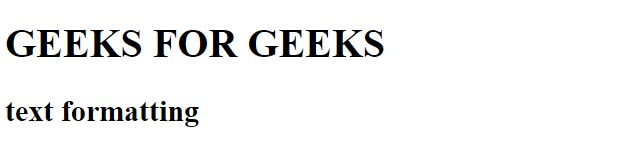Example 5: This example illustrates the use of the text-indent property.

## html

 `` `<``html``>`   `<``head``>` `    ``<``style``>` `        ``h2 {` `            ``text-indent: 80px;` `        ``}` `    ``` ``   `<``body``>` `    ``<``h1``>` `        ``GEEKS FOR GEEKS` `    ``` `    ``<``h2``>` `        ``This is text formatting properties.<``br` `/>` `        ``Text indentation property is used to` `        ``indent the first line of the paragraph.` `    ``` ``   ``

Output: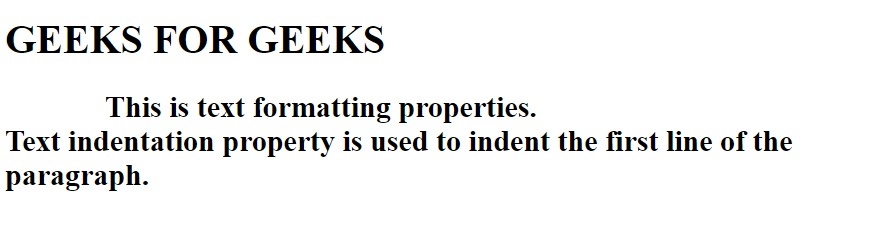Example 6: This example illustrates the use of the letter-spacing property.

## html

 `` `<``html``>`   `<``head``>` `    ``<``style``>` `        ``h2 {` `            ``letter-spacing: 4px;` `        ``}` `    ``` ``   `<``body``>` `    ``<``h1``>` `        ``GEEKS FOR GEEKS` `    ``` `    ``<``h2``>` `        ``This is text formatting properties.` `    ``` ``   ``

Output: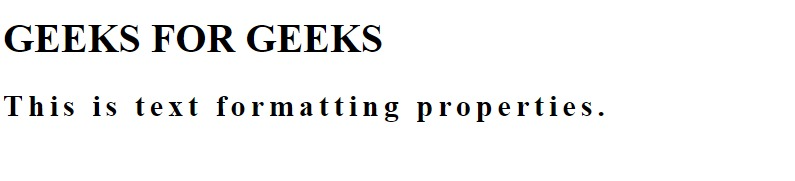Example 7: This example illustrates the use of the line-height property.

## html

 `` `<``html``>`   `<``head``>` `    ``<``style``>` `        ``h2 {` `            ``line-height: 40px;` `        ``}` `    ``` ``   `<``body``>` `    ``<``h1``>` `        ``GEEKS FOR GEEKS` `    ``` `    ``<``h2``>` `        ``This is text formatting properties.<``br` `/>` `        ``This property is used to set the` `        ``space between the lines.` `    ``` ``   ``

Output: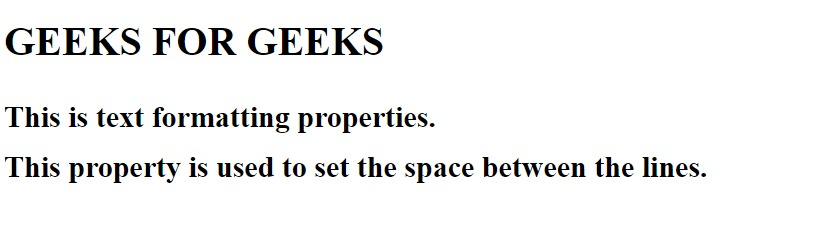Example 8: This example illustrates the use of the direction property.

## html

 `` `<``html``>` `    ``<``head``>` `        ``<``style``>` `            ``h2 {` `                ``direction: rtl;` `                ``text-align: center;` `            ``}` `        ``` `    ``` `    ``<``body``>` `        ``<``h1``>` `            ``GEEKS FOR GEEKS` `        ``` `        ``<``h2``>` `            ``<``bdo` `dir``=``"rtl"``>` `                ``This is text formatting properties.` `            ``` `        ``` `    ``` ``

Output: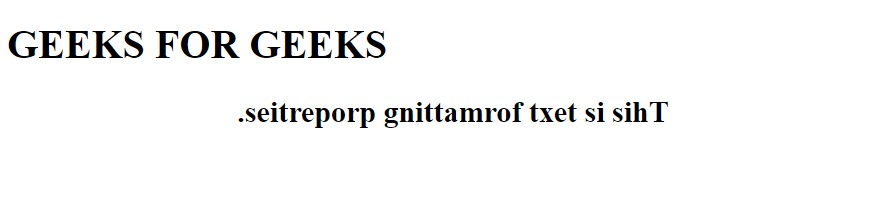Example 9: This example illustrates the use of the text-shadow property.

## html

 `` `<``html``>`   `<``head``>` `    ``<``style``>` `        ``h1 {` `            ``text-shadow: 3px 1px blue;` `        ``}` `    ``` ``   `<``body``>` `    ``<``h1``>` `        ``GEEKS FOR GEEKS` `    ``` `    ``<``h2``>` `        ``This is text formatting properties.` `    ``` ``   ``

Output: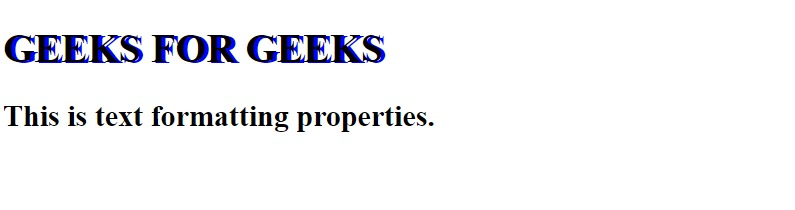Example 10: This example illustrates the use of the word-spacing property.

## html

 `` `<``html``>`   `<``head``>` `    ``<``style``>` `        ``h2 {` `            ``word-spacing: 15px;` `        ``}` `    ``` ``   `<``body``>` `    ``<``h1``>` `        ``GEEKS FOR GEEKS` `    ``` `    ``<``h2``>` `        ``This is text formatting properties.` `    ``` ``   ``

Output: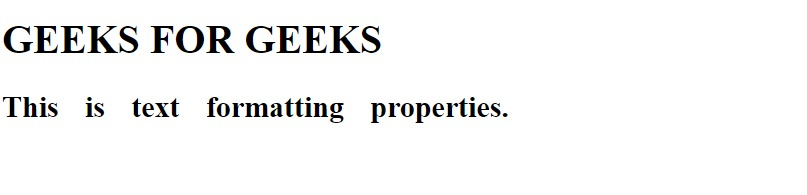Supported Browser: